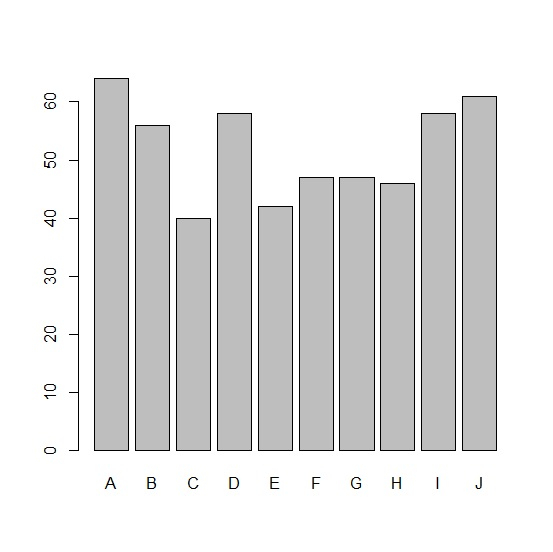# How to create barplot for some top values in an R data frame?

R ProgrammingServer Side ProgrammingProgramming

To create barplot for some top values in an R data frame, we can subset the required top values inside the barplot function.

For example, if we have a data frame called df that contains a categorical column say C and a numerical column for frequency defined as F then the barplot for top five values can be created by using the below mentioned command −

barplot(df$F[1:5],names.arg=df$C[1:5])

## Example

Following snippet creates a sample data frame −

Category<-LETTERS[1:20]
count<-rpois(20,50)
df<-data.frame(Category,count)
df

## Output

The following dataframe is created −

Category count
1  A    64
2  B    56
3  C    40
4  D    58
5  E    42
6  F    47
7  G    47
8  H    46
9  I    58
10 J    61
11 K    55
12 L    46
13 M    54
14 N    64
15 O    53
16 P    43
17 Q    58
18 R    42
19 S    44
20 T    58

In order to create a barplot for top ten values in df, add the following code to the above snippet −

Category<-LETTERS[1:20]
count<-rpois(20,50)
df<-data.frame(Category,count)
barplot(df$count[1:10],names.arg=df$Category[1:10])

## Output

If you execute all the above given snippets as a single program, it generates the following output −